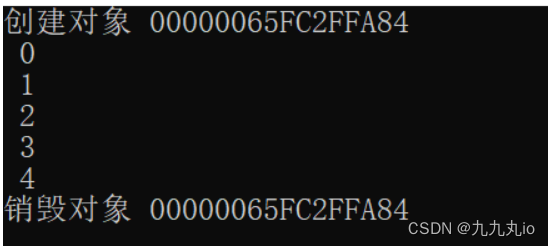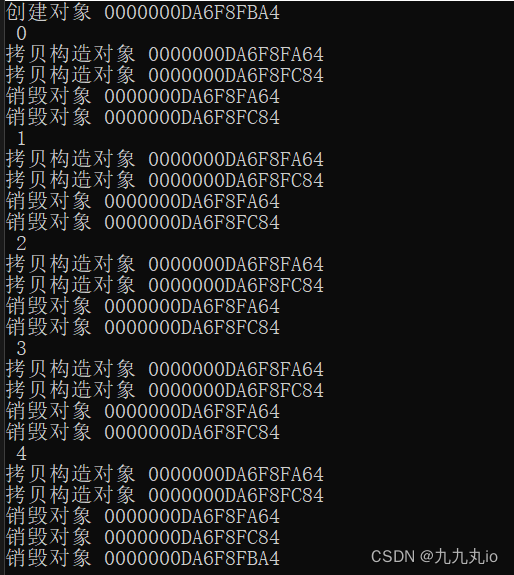> 文档中心 > C++之运算符重载(前置++和后置++)

# C++之运算符重载(前置++和后置++)

++a表示取a的地址，增加它的内容，然后把值放在寄存器中；

a++表示取a的地址，把它的值装入寄存器，然后增加内存中的a的值；

``class INT{private:int value;public:void Print(){cout << " " << value << endl;}INT(int v=0) :value(v){cout << "创建对象 " << this << endl;}~INT(){cout << "销毁对象 " << this << endl;}INT(const INT& mc) :value(mc.value){cout << "拷贝构造对象 " << this <value += 1;return *this;}const INT  operator++(int)  //后置++{INT od = *this;++*this;return od;//return INT(this->value++);}operator int const() { return value; }//重载了一个整型强转};``

1. 返回类型不同
2. 形参不同
3. 效率不同

1.返回值类型的区别

``int main()     {  INT a;      (a++)++;  //编译错误  ++(a++);  //编译错误  a++ = 1;   //编译错误  (++a)++;  //OK  ++(++a);  //OK  ++a = 1;   //OK     }    ``

++的类型是const INT，自然不能对它进行前置++、后置++、赋值等操作。

++a的类型是INT&，当然可以对它进行前置++、后置++、赋值等操作

a++的返回类型为什么要是const对象呢？

1. 如果不是const对象，a(++)++这样的表达式就可以通过编译。但是，其效果却违反了我们的直觉 。a其实只增加了1，因为第二次自增作用在一个临时对象上。
2. 另外，对于内置类型，(i++)++这样的表达式是不能通过编译的。自定义类型的操作符重载，应该与内置类型保持行为一致 。

a++的返回类型如果改成非const对象，肯定能通过编译，但是我们最好不要这样做。

++a的返回类型为什么是引用呢？

2.形参的区别

3.效率的区别

``const INT  operator++(int)  //后置++{INT old = *this;++*this;  //利用前置++return old;//return INT(this->value++);  //此方法也可以}``

• ++i  创建一对象，销毁一对象
``int main(){for (INT i = 0; i < 5;++i){i.Print();}}``•  i++  后置++返回的操作之前的一个副本值，需要不断的拷贝和销毁
``int main(){for (INT i = 0; i < 5;i++){i.Print();}}``